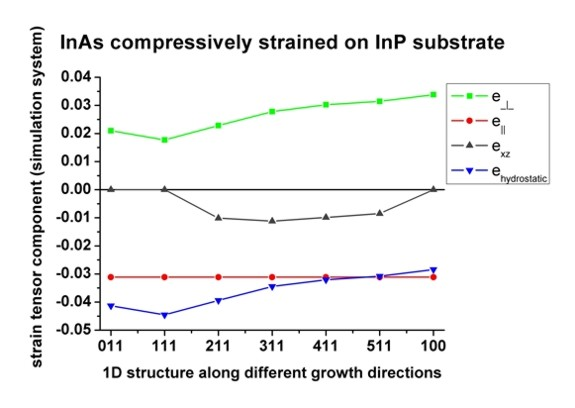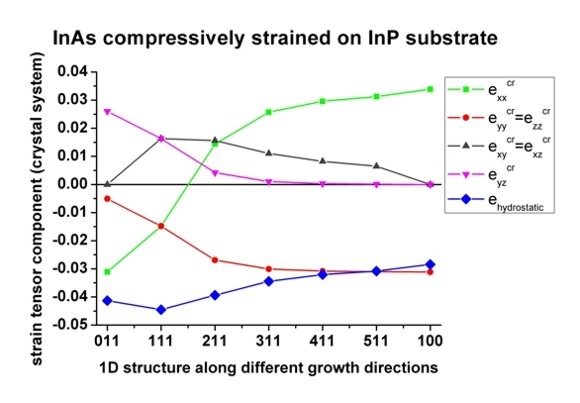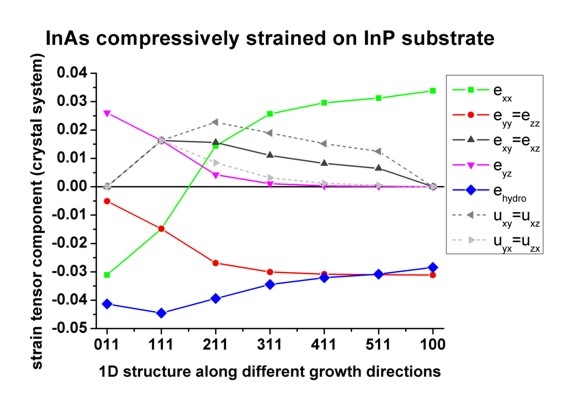nextnano.com  GUI: nextnanomat  Tool: nextnano++  Tool: nextnano³  Tool: nextnano.NEGF  Download | Search | Copyright | News | Publications  * password protected nextnano³ software1D strain

# nextnano3 - Tutorial

## Strain and displacement tensors along different growth directions

Author: Stefan Birner

If you want to obtain the input files that are used within this tutorial, please contact stefan.birner@nextnano.de.
```-> 1DstrainN11.in / *_nnp.in - ```input file for the nextnano3 and nextnano++ software``` -> 2DstrainN11.in / *_nnp.in - ```input file for the nextnano3 and nextnano++ software``` -> 3DstrainN11.in / *_nnp.in - ```input file for the nextnano3 and nextnano++ software```  ```

## AlAs/InAs/AlAs structure on InP

• This input file simulates a InP/InAs/AlAs structure grown pseudomorphically on InP:``` 1DstrainN11.in```
The structure is grown pseudomorphically on InP, i.e. the InAs is compressively strained, the AlAs is tensilely strained. The growth direction [N11] is along x, the interfaces are in the (y,z) plane.
• This tutorial examines the strain tensors in the crystal and simulation coordinate system as well as the corresponding displacement tensors.
The following parameters are used:
 InP (substrate) InAs AlAs Lattice constant a (nm) 0.58697 0.60583 0.56611 Elastic constant c11 (GPa) 101.1 83.29 125.0 Elastic constant c12 (GPa) 56.1 45.26 53.4 Elastic constant c44 (GPa) 45.6 39.59 54.20

•  growth direction
(y direction along )
``` \$domain-coordinates  ...  hkl-x-direction-zb     = 1 0 0  ! Miller indices of x coordinate axis [1 0 0]  hkl-y-direction-zb     = 0 1 0  ! Miller indices of y coordinate axis [0 1 0]  growth-coordinate-axis = 1 0 0  ... ```
The strain tensors in the crystal and simulation system are identical. Off-diagonal strain components eij are zero. The hydrostatic strain is defined as the trace of the strain tensor (ehydro=exx+eyy+ezz). It is useful to talk about parallel strain (e||=eyy=ezz) and perpendicular (e_|_=exx) strain with respect to the interface coordinate system.
Biaxial strain (in plane of interface):
InAs: e|| = eyy = ezz = ( asubstrate - alayer ) / alayer = -0.0311308   (3.1 % lattice mismatch)
Uniaxial strain (perpendicular to interface):
InAs: e_|_ = exx = - D100 e|| = - 2 (c12/c11) e|| = 0.0338332
For  growth direction the strain tensors and the displacement tensors coincide.
  exx=e_|_ eyy=ezz=e|| ehydro InAs 0.338332E-001 -0.311308E-001 -0.284285E-001 AlAs -0.314829E-001 0.368480E-001 0.422130E-001

•  growth direction
(y direction along [01-1])
``` \$domain-coordinates  ...  hkl-x-direction-zb     = 0 1  1 ! Miller indices of x coordinate axis [1 0 0]  hkl-y-direction-zb     = 0 1 -1 ! Miller indices of y coordinate axis [0 1 0]  growth-coordinate-axis = 1 0 0  ... ```The strain tensors in the crystal and simulation system are not identical any more. Off-diagonal strain components eij are zero apart from eyzcr. The hydrostatic strain is independent of coordinate system.
Biaxial strain (in plane of interface):
InAs: e||=eyysim = ezzsim = ( asubstrate - alayer ) / alayer = -0.0311308   (same as for )
Uniaxial strain (perpendicular to interface):
InAs: e_|_ =exxsim = - D011e|| = - (c11+3c12-2c44) / (c11+c12+2c44) e|| = 0.0209642
Crystal system: InAs: eyycr = ezzcr = - ??? e|| = -0.00508332
InAs: eyzcr = - (c11+2c12) / (c11+c12+2c44) e|| = 0.0260475
For  growth direction the strain tensors and the displacement tensors coincide.
Here it is interesting to note that exxcr = e||.
  exxsim=e_|_ eyysim=ezzsim=e|| ehydro InAs 0.209642E-001 -0.311308E-001 -0.412975E-001 AlAs -0.227152E-001 0.368480E-001 0.509807E-001
  exxcr eyycr=ezzcr eyzcr InAs -0.311308E-001 -0.508332E-002 0.260475E-001 AlAs 0.368480E-001 0.706638E-002 -0.297816E-001

•  growth direction
(y direction along [01-1])
``` \$domain-coordinates  ...  hkl-x-direction-zb     = 1 1  1 ! Miller indices of x coordinate axis [1 0 0]  hkl-y-direction-zb     = 0 1 -1 ! Miller indices of y coordinate axis [0 1 0]  growth-coordinate-axis = 1 0 0  ... ```The strain tensors in the crystal and simulation system are not identical. Off-diagonal strain components eij are still zero in the simulation system but not in the crystal system.
Simulation system:
Biaxial
strain (in plane of interface):
InAs: e||=eyysim = ezzsim = ( asubstrate - alayer ) / alayer = -0.0311308   (same as for )
Uniaxial strain (perpendicular to interface):
InAs: e_|_ =exxsim = - D111e|| = - 2(c11+2c12-2c44) / (c11+2c12+4c44) e|| = 0.0177374
Crystal system: InAs: exxcr = eyycr = ezzcr = - (-4c44) / (c11+2c12+4c44) e|| = -0.0148414
InAs: exycr = exzcr = eyzcr = - (c11+2c12) / (c11+2c12+4c44) e|| = 0.0162894

For  growth direction the strain tensors and the displacement tensors coincide.
  exxsim=e_|_ eyysim=ezzsim=e|| ehydro InAs 0.177374E-001 -0.311308E-001 -0.445243E-001 AlAs -0.202721E-001 0.368480E-001 0.534238E-001
  exxcr=eyycr=ezzcr exycr=exzcr=eyzcr InAs -0.148414E-001 0.162894E-001 AlAs 0.178079E-001 -0.190400E-001

•  growth direction
(y direction along [01-1])
``` \$domain-coordinates  ...  hkl-x-direction-zb     = 2 1  1 ! Miller indices of x coordinate axis [1 0 0]  hkl-y-direction-zb     = 0 1 -1 ! Miller indices of y coordinate axis [0 1 0]  growth-coordinate-axis = 1 0 0  ... ```Off-diagonal strain components eij are not zero any more in the simulation system. For  growth direction the strain tensors and the displacement tensors do not coincide any more because the displacement tensors are not symmetric any more. The diagonal entries still coincide eii=uii.
Biaxial strain (in plane of interface):
InAs: e||=eyysim = ezzsim = ( asubstrate - alayer ) / alayer = -0.0311308   (same as for )
Uniaxial strain (perpendicular to interface):
InAs: e_|_ =exxsim = - D211e|| = - (D211=???) e|| = 0.0228540
  exxsim=e_|_ eyysim=ezzsim=e|| exzsim ehydro uzxsim=duz/dx uxzsim=dux/dz InAs 0.228540E-001 -0.311308E-001 -0.101187E-001 -0.394077E-001 -0.202373E-001 = 2exzsim 0 AlAs -0.234699E-001 0.368480E-001 0.623832E-002 0.502260E-001 0.124766E-001 = 2exzsim 0
  exxcrr eyycr=ezzcr exycr=exzcr eyzcr uxycr=uxzcr uyxcr=uzxcr uyzcr=uzycr InAs 0.143990E-001 -0.269034E-001 0.156100E-001 0.422749E-002 0.227649E-001 0.845498E-002 0.422749E-002 = eyzcr AlAs -0.924548E-002 0.297358E-001 -0.186356E-001 -0.711220E-002 -0.230467E-001 -0.142244E-001 -0.711220E-002 = eyzcr

One can see that the displacement tensor for the simulation system is rather nice as the following off-diagonal components are zero:
- uxysim, uxzsim, uyzsim  are always zero for 1D structures grown along the x axis of the simulation system.
- uyxsim, uzysim  are zero in this particular example.
Here one should recall the definiton of the strain tensor: eij = 1/2 (uij + uji) = 1/2 (dui/dj + duj/di)
The strain tensor is thus always symmetric but the displacement tensor only for growth directions along , , . In fact, for these three directions it is also diagonal in the simulation coordinate system. In this particular example, uxycr is not equal to uyxcr.

•  growth direction
(y direction along [01-1])
``` \$domain-coordinates  ...  hkl-x-direction-zb     = 3 1  1 ! Miller indices of x coordinate axis [1 0 0]  hkl-y-direction-zb     = 0 1 -1 ! Miller indices of y coordinate axis [0 1 0]  growth-coordinate-axis = 1 0 0  ... ```See comments for  growth direction

Biaxial strain (in plane of interface):
InAs: e||=eyysim = ezzsim = ( asubstrate - alayer ) / alayer = -0.0311308   (same as for )
Uniaxial strain (perpendicular to interface):
InAs: e_|_ =exxsim = - D311e|| = - (D311=???) e|| = 0.0277907
  exxsim=e_|_ eyysim=ezzsim=e|| exzsim ehydro uzxsim=duz/dx uxzsim=dux/dz InAs 0.277907E-001 -0.311308E-001 -0.111833E-001 -0.344710E-001 -0.223666E-001 = 2exzsim 0 AlAs -0.267795E-001 0.368480E-001 0.714093E-002 0.469164E-001 0.142819E-001 = 2exzsim 0
  exxcrr eyycr=ezzcr exycr=exzcr eyzcr uxycr=uxzcr uyxcr=uzxcr uyzcr=uzycr InAs 0.257044E-001 -0.300877E-001 0.110373E-001 0.104316E-002 0.189451E-001 0.312949E-002 0.104316E-002 = eyzcr AlAs -0.207193E-001 0.338179E-001 -0.141397E-001 -0.303010E-002 -0.191891E-001 -0.909030E-002 -0.303010E-002 = eyzcr

•  growth direction
(y direction along [01-1])
``` \$domain-coordinates  ...  hkl-x-direction-zb     = 4 1  1 ! Miller indices of x coordinate axis [1 0 0]  hkl-y-direction-zb     = 0 1 -1 ! Miller indices of y coordinate axis [0 1 0]  growth-coordinate-axis = 1 0 0  ... ```See comments for  growth direction

Biaxial strain (in plane of interface):
InAs: e||=eyysim = ezzsim = ( asubstrate - alayer ) / alayer = -0.0311308   (same as for )
Uniaxial strain (perpendicular to interface):
InAs: e_|_ =exxsim = - D411e|| = - (D411=???) e|| = 0.0302133
  exxsim=e_|_ eyysim=ezzsim=e|| exzsim ehydro uzxsim=duz/dx uxzsim=dux/dz InAs 0.302133E-001 -0.311308E-001 -0.986895E-002 -0.320484E-001 -0.197379E-001 = 2exzsim 0 AlAs -0.285575E-001 0.368480E-001 0.646539E-002 0.451384E-001 0.129308E-001 = 2exzsim 0
  exxcrr eyycr=ezzcr exycr=exzcr eyzcr uxycr=uxzcr uyxcr=uzxcr uyzcr=uzycr InAs 0.296003E-001 -0.308243E-001 0.820438E-002 0.306496E-003 0.151828E-001 0.122598E-002 0.306496E-003 = eyzcr AlAs -0.253540E-001 0.352462E-001 -0.109788E-001 -0.160176E-002 -0.155505E-001 -0.640704E-002 -0.160176E-002 = eyzcr

•  growth direction
(y direction along [01-1])
``` \$domain-coordinates  ...  hkl-x-direction-zb     = 5 1  1 ! Miller indices of x coordinate axis [1 0 0]  hkl-y-direction-zb     = 0 1 -1 ! Miller indices of y coordinate axis [0 1 0]  growth-coordinate-axis = 1 0 0  ... ```See comments for  growth direction

Biaxial strain (in plane of interface):
InAs: e||=eyysim = ezzsim = ( asubstrate - alayer ) / alayer = -0.0311308   (same as for )
Uniaxial strain (perpendicular to interface):
InAs: e_|_ =exxsim = - D511e|| = - (D511=???) e|| =  0.0314570
  exxsim=e_|_ eyysim=ezzsim=e|| exzsim ehydro uzxsim=duz/dx uxzsim=dux/dz InAs 0.314570E-001 -0.311308E-001 -0.847870E-002 -0.308047E-001 -0.169574E-001 = 2exzsim 0 AlAs -0.295219E-001 0.368480E-001 0.563906E-002 0.441740E-001 0.112781E-001 = 2exzsim 0
  exxcrr eyycr=ezzcr exycr=exzcr eyzcr uxycr=uxzcr uyxcr=uzxcr uyzcr=uzycr InAs 0.312618E-001 -0.310333E-001 0.648318E-002 0.975671E-004 0.124785E-001 0.487835E-003 0.975671E-004 = eyzcr AlAs -0.275593E-001 0.358666E-001 -0.889403E-002 -0.981324E-003 -0.128815E-001 -0.490662E-002 -0.981324E-003 = eyzcr

•  growth direction
(y direction along [-111])
``` \$domain-coordinates  ...  hkl-x-direction-zb     =  3 2 1 ! Miller indices of x coordinate axis [1 0 0]  hkl-y-direction-zb     = -1 1 1 ! Miller indices of y coordinate axis [0 1 0]  growth-coordinate-axis = 1 0 0  ... ```
Biaxial strain (in plane of interface):
InAs: e||=eyysim = ezzsim = ( asubstrate - alayer ) / alayer = -0.0311308   (same as for )
Uniaxial strain (perpendicular to interface):
InAs: e_|_ =exxsim = - D321e|| = - (D321=???) e|| = 0.0223345
  exxsim=e_|_ eyysim=ezzsim=e|| exysim exzsim ehydro uyxsim=duy/dx uzxsim=duz/dx uxysim=uxzsim InAs 0.223345E-001 -0.311308E-001 -0.871721E-002 0.139475E-002 -0.399271E-001 -0.174344E-001 = 2exysim 0.278950E-002 = 2exzsim 0 AlAs -0.232538E-001 0.368480E-001 0.528954E-002 -0.515392E-003 0.504421E-001 0.105791E-001 = 2exysim -0.103078E-002 = 2exzsim 0
  exxcrr eyycr ezzcr exycr exzcr eyzcr InAs 0.116554E-001 -0.221557E-001 -0.294269E-001 0.209935E-001 0.968697E-002 0.394774E-002 AlAs -0.681357E-002 0.232809E-001 0.339748E-001 -0.247292E-001 -0.115866E-001 -0.626491E-002
  uxycr uyxcr uxzcr uzxcr uyzcr uzycr InAs 0.285242E-001 0.134627E-001 0.142621E-001 0.511185E-002 0.448757E-002 0.340790E-002 AlAs -0.291077E-001 -0.203506E-001 -0.145538E-001 -0.861942E-002 -0.678354E-002 -0.574628E-002

• Simulation system
Now we plot the above results for [N11] growth directions. Note that  is identical to ["infinity"11] growth direction. All plotted quantities are given in the simulation system.For ,  and , the off-diagonal strain tensor component exzsim is zero. For the "high symmetry growth directions" the strain and displacement tensors are diagonal. (But this does not necessarily mean that shear strain is absent. Shear strain is only absent if the strain tensor is diagonal in the crystal system, i.e. only for  growth direction.)
The hydrostatic strain ehy=Tr(eij) is always negative because we have a volume reduction (compressive strain). It has the largest value in magnitude for  and the smallest in magnitude for . It shows exactly the same behaviour as the curve for e_|_sim, i.e. e_|_sim is a measure for the volume deformation in the growth direction.
The strain in the plane of interface e||sim is constant for all growth directions.
Note that the highest value for offdiagonal strain exz occurs for  growth direction (highest shear strain of any orientation).
• Crystal system
Now we plot the above results for [N11] growth directions. Note that  is identical to ["infinity"11] growth direction. All plotted quantities are given in the crystal system.The hydrostatic strain ehy=Tr(eij) is identical to the plot of the simulation system. (The trace of a matrix is independent of coordinate system.) It is always negative because we have a volume reduction (compressive strain). It has the largest value in magnitude for  and the smallest in magnitude for .
For  growth direction, exxcr=eyycr=ezzcr and exycr=exzcr=eyzcr.
All off-diagonal components are zero only for  growth direction (no shear strain). For all other orientations we have shear strain. Shear strain (with respect to the crystal system) is responsible for piezoelectric effects.
The off-diagonal components exycr=exzcr are zero for  growth direction but the off-diagonal component eyzcr is not.
• Here the same picture is plotted again (crystal system) but this time including the important components of the displacement tensor that are responsible for the fact that the displacement tensor is not symmetric any more for , ,  and .
The strain tensor is defined as: eij = 1/2 (uij + uji) = 1/2 (dui/dj + duj/di)
Thus: exycr = 1/2 (uxycr+uyxcr).

For the high symmetry growth direction , ,  the displacement tensor is symmetric because

 uxycr=uyxcr=exycr.• Strain has important effects:
- piezoelectric fields: See Tutorial on piezoelectricity.
- shifts and splittings of conduction and valence bands: See Tutorial on deformation potentials.
- strain changes the k.p Hamiltonian
• Strain can be used to tailor the electronic and optical properties of heterostructures.
• Please help us to improve our tutorial! Send comments to``` support [at] nextnano.com```.